# Notebook 2.3 - Studying the London Underground¶

### Part 1 - Drawing the London Underground network¶

In :
import networkx as nx
import pandas as pd
import matplotlib.pyplot as plt

In :
stations = pd.read_csv('datasets/lu_stations.csv')
stations

Out:
id latitude longitude name zone
0 1 51.5028 -0.2801 Acton Town 3.0
1 8 51.5653 -0.1353 Archway 2.5
2 9 51.6164 -0.1331 Arnos Grove 4.0
3 10 51.5586 -0.1059 Arsenal 2.0
4 11 51.5226 -0.1571 Baker Street 1.0
... ... ... ... ... ...
139 296 51.5120 -0.2239 White City 2.0
140 297 51.5492 -0.2215 Willesden Green 2.5
141 303 51.5975 -0.1097 Wood Green 3.0
142 301 51.6070 0.0341 Woodford 4.0
143 302 51.6179 -0.1856 Woodside Park 4.0

144 rows × 5 columns

In :
links = pd.read_csv('datasets/lu_links.csv')

Out:
station1 station2 line time
0 1 234 10 4
1 1 265 10 4
2 8 124 9 3
3 8 264 9 2
4 9 31 10 3
... ... ... ... ...
164 257 258 9 2
165 261 302 9 3
166 266 303 10 2
167 279 285 7 2
168 288 302 9 1

169 rows × 4 columns

No surprises here - let's now convert these into a graph.

In :
G = nx.Graph()

nx.draw(G)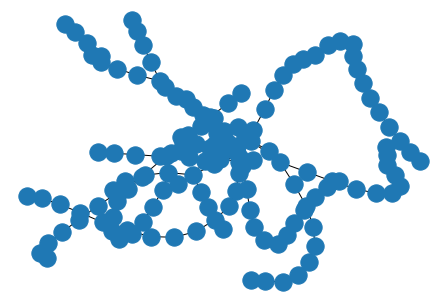We have such a large number of nodes, and this ends up being a very busy graph. We can amend the way that the nodes are plotted, so that it looks a bit nicer. We can do this using the node_size parameter.

In :
nx.draw(G, node_size = 6)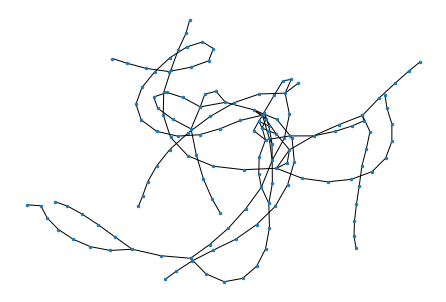But it remains a bit difficult to see - what if we could we make it a bit bigger?

This is possible using a few more advanced matplotlib features. You see, in every new cell we are creating a new instance of a matplotlib chart. Thanks to the pyplot library within matplotlib, the chart creation is quite similar to the one found in Matlab - so some concepts might look familiar.

To modify the size of the figure, we simply have to initialise the chart ourselves, usiing the plt.figure() command, and then specify its size using the figsize command.

If you want to more help with the transition from Matlab to Python, you can read this very helpful guide, or follow this DataCamp course.

In :
plt.figure(figsize=(16,10))
nx.draw(G, node_size = 40)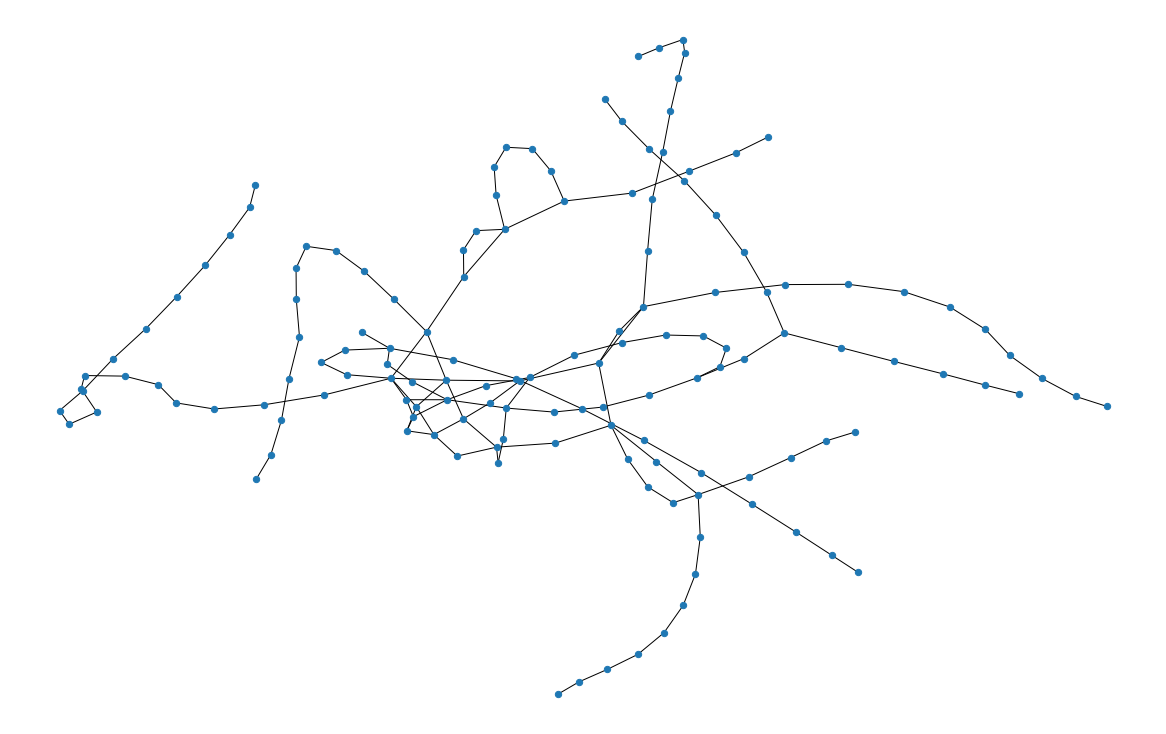Much better, but now that we have a better look at it, this certainly doesn't look anything like the London Tube.

Ah! But of course! We forgot to add the coordinates.

In :
plt.figure(figsize=(16,10))

coords = list(zip(stations['longitude'],stations['latitude']))
pos = dict(zip(stations['id'], coords))
nx.draw(G,pos,node_size = 40)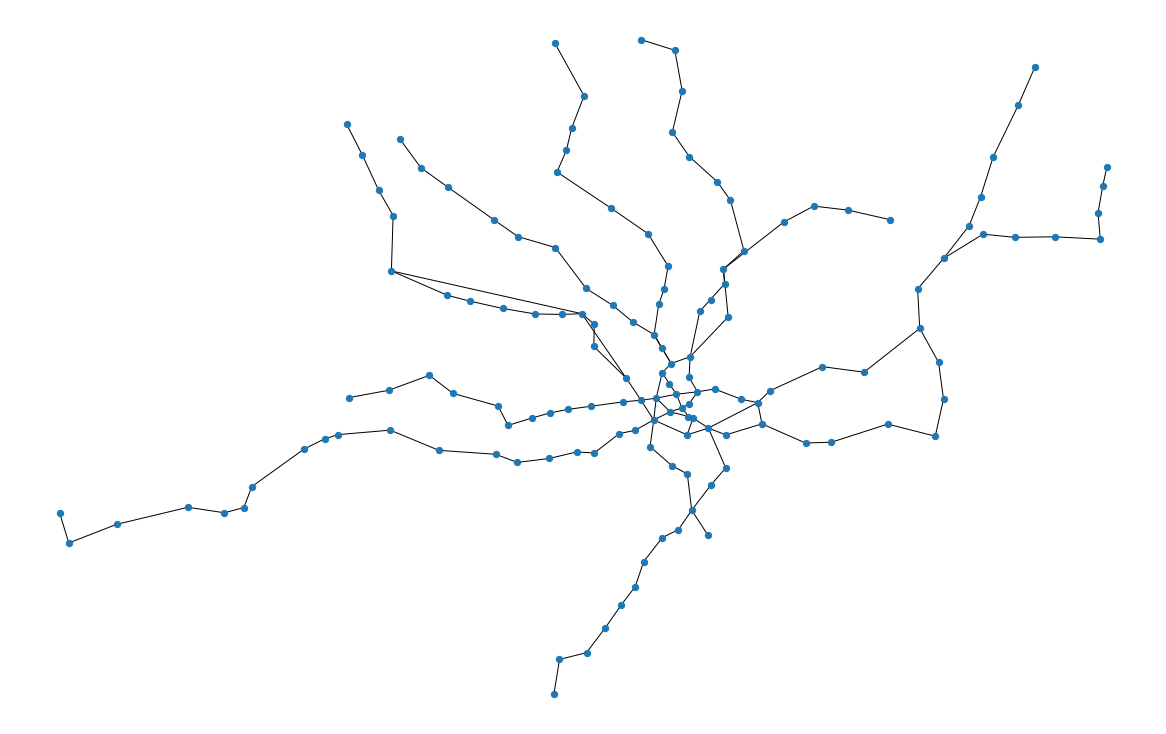### Part 2 - Extraction of network subgraphs¶

What if we wanted to only illustrate the subgraph of the network that lies within Zones 1?

We can do that easily using using the zone column in the stations dataframe - note that the authors of that list chose use "half" values to denote stations that lie in two zones at the same time. Therefore Archway station is described in being in zone 2.5, when in official maps it is placed on the boundaries of zones 2 and 3.

Therefore, if we want to obtain all the nodes that are found in zone 1, we would really have to obtain the stations with a zone value of <2 - it we used <=1 to filter the list, we would have excluded stations that lie in the zone boundary, such as Earls Court.

In :
stations_z1 = pd.read_csv('datasets/lu_stations.csv')
stations_z1 = stations_z1[stations_z1['zone']<2]
len(stations_z1)

Out:
36

We filtered the stations using a condition applied on the zone columnn. This effectively says:

"Look at the zone column within the stations_z1 dataframe, and select the rows where its value is less than 2. Now return a new dataframe, that contains only these rows".

We can now proceed to filter the stations. The stations dataframe does not contain any information on zones, but we can do this by filtering the list by checking whether both stations in each edge are found within out filtered list of Zone 1 station.

To do this, we first create a list of all "allowed" node IDs. We then filter the list be exluding any link whose endpoints do not belong in Zone 1.

In :
allowed_stations = list(stations_z1['id'])


Out:
57

We have now the list down to 57 nodes, which have an allowed station in the station1 column. Let's now apply the filter to station2.

In :
links_z1 = links_z1.loc[links_z1['station2'].isin(allowed_stations)]

Out:
54

Let's now visualise the part of the network:

In :
G_z1 = nx.Graph()

plt.figure(figsize=(16,10))
coords = list(zip(stations_z1['longitude'],stations_z1['latitude']))
pos = dict(zip(stations_z1['id'], coords))
nx.draw(G_z1, pos, node_size = 60)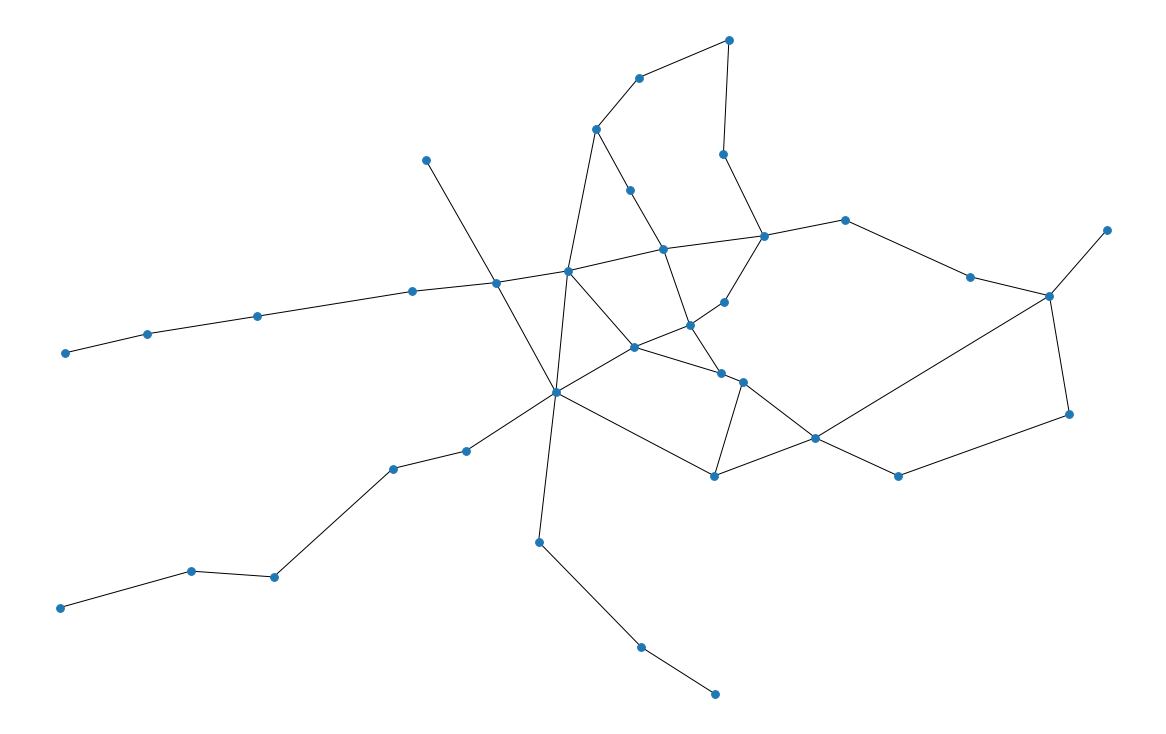### Part 3 - Obtaining centrality metrics¶

We can now add a list of our centralities.

I am going to use a lambda function to add station names into a column, based on dictionary and the value of the ID column. There are much easier ways to achieve this, but I wanted to take this opportunity to show you the lambda feature in action.

In :
dict_names = dict(zip(stations['id'],stations['name']))

In :
centralities = pd.DataFrame()
centralities['ID'] = G.nodes()
centralities['Names'] = centralities["ID"].map(lambda x:dict_names[x])
centralities['degree_centr'] = nx.degree_centrality(G).values()
centralities['closeness_centr'] = nx.closeness_centrality(G).values()
centralities['betweenness_centr'] = nx.betweenness_centrality(G).values()
centralities['eigenvector_centr'] = nx.eigenvector_centrality(G).values()


Let us now obtain our "Top 10" lists.

In :
centralities.sort_values(by='degree_centr', ascending=False).head(10).reset_index()[['Names','degree_centr']]

Out:
Names degree_centr
0 Green Park 0.041958
1 Oxford Circus 0.034965
2 Waterloo 0.034965
3 Leicester Square 0.027972
4 Bond Street 0.027972
5 Euston 0.027972
6 Finsbury Park 0.027972
8 Stockwell 0.027972
In :
centralities.sort_values(by='closeness_centr', ascending=False).head(10).reset_index()[['Names','closeness_centr']]

Out:
Names closeness_centr
0 Green Park 0.135417
1 Oxford Circus 0.133023
2 Bond Street 0.129882
3 Westminster 0.127565
4 Warren Street 0.126102
7 Hyde Park Corner 0.123064
8 Victoria 0.122118
9 Waterloo 0.122014
In :
centralities.sort_values(by='betweenness_centr', ascending=False).head(10).reset_index()[['Names','betweenness_centr']]

Out:
Names betweenness_centr
0 Green Park 0.483804
1 Euston 0.372402
2 Oxford Circus 0.340179
3 Warren Street 0.307614
4 Bond Street 0.305722
5 Bank & Monument 0.282445
6 Waterloo 0.250051
7 King's Cross St. Pancras 0.248839
8 Camden Town 0.240914
9 Hyde Park Corner 0.221609
In :
centralities.sort_values(by='eigenvector_centr', ascending=False).head(10).reset_index()[['Names','eigenvector_centr']]

Out:
Names eigenvector_centr
0 Green Park 0.425429
1 Oxford Circus 0.419025
3 Bond Street 0.286223
4 Leicester Square 0.265054
6 Charing Cross 0.219205
7 Westminster 0.200704
8 Warren Street 0.171072
9 Embankment 0.155901

The overwhelming message here is that Green Park is definitely important!

### Part 4 - Generation of centrality visualisations¶

Let's now visualise these values:

In :
plt.figure(figsize=(16,10))
coords = list(zip(stations['longitude'],stations['latitude']))
pos = dict(zip(stations['id'], coords))
nx.draw(G, pos, with_labels = False, node_color = list(centralities['degree_centr']))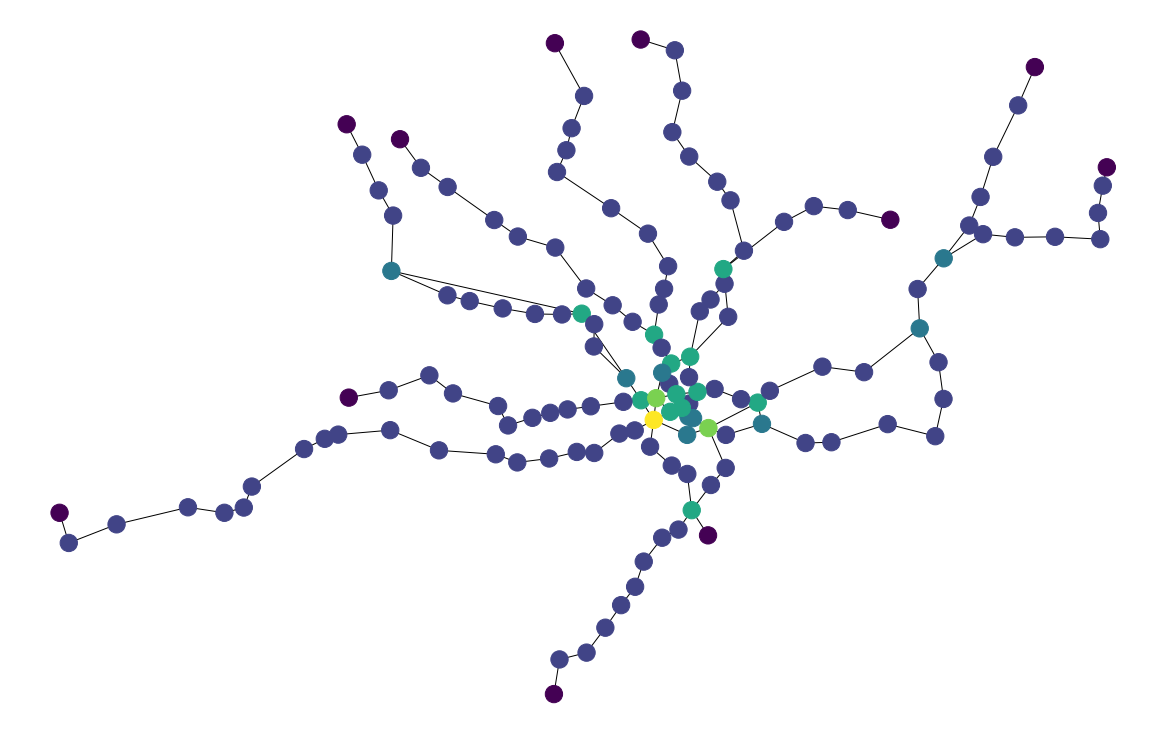In :
plt.figure(figsize=(16,10))
coords = list(zip(stations['longitude'],stations['latitude']))
pos = dict(zip(stations['id'], coords))
nx.draw(G, pos, with_labels = False, node_color = list(centralities['betweenness_centr']))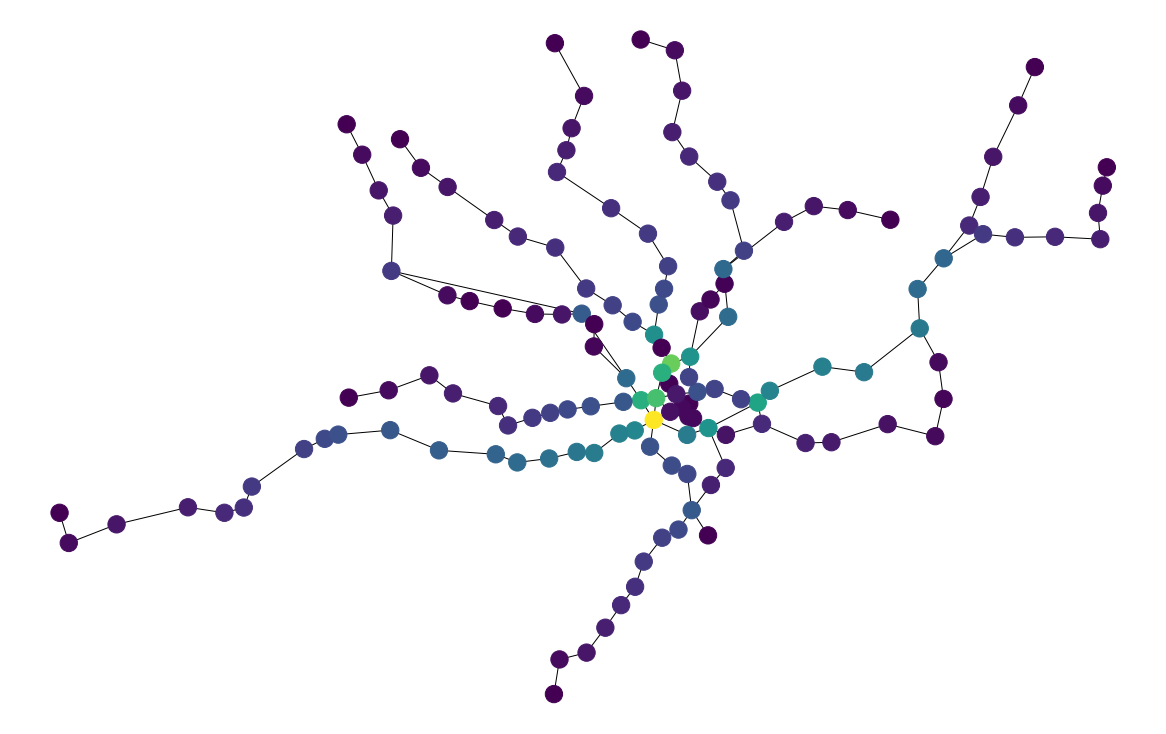### Part 5 - Using K-Means to obtain zones¶

At this point we will apply the K-Means algorithm approach in order to split the network into zones. I would strongly advise that you pause this notebook for the time being, and instead have a look at the next one in this serios (2.4 - Kmeans clustering), which introduces the fundamentals of this method in more detail.

You can return back to this notebook once you had a look on that.

To proceed, we import the KMeans cluster from sklearn and the numpy package.

In :
from sklearn.cluster import KMeans
import numpy as np


The Kmeans algorithm can work with any dataset - in our case we will simply apply it to an array of the station coordinates.

In :
coord = np.array(list(zip(stations['longitude'],stations['latitude'])))

In :
model = KMeans(n_clusters=10)
model.fit(coord)
clust_pred = model.predict(coord)


We use the same code as in Notebook 2.3 to plot the cluster memberships.

In :
plot_size   = 20
plot_width  = 10
plot_height = 10

params = {'legend.fontsize': 'large',
'figure.figsize': (plot_width,plot_height),
'axes.labelsize': plot_size,
'axes.titlesize': plot_size,
'xtick.labelsize': plot_size*0.5,
'ytick.labelsize': plot_size*0.50,
plt.rcParams.update(params)

plt.scatter(coord[:, 0],
coord[:, 1],
c = clust_pred,
s=plot_size*2,
cmap='Accent')

centers = model.cluster_centers_

plt.scatter(centers[:, 0],
centers[:, 1],
c = 'red',
s=plot_size*10,
alpha=0.5);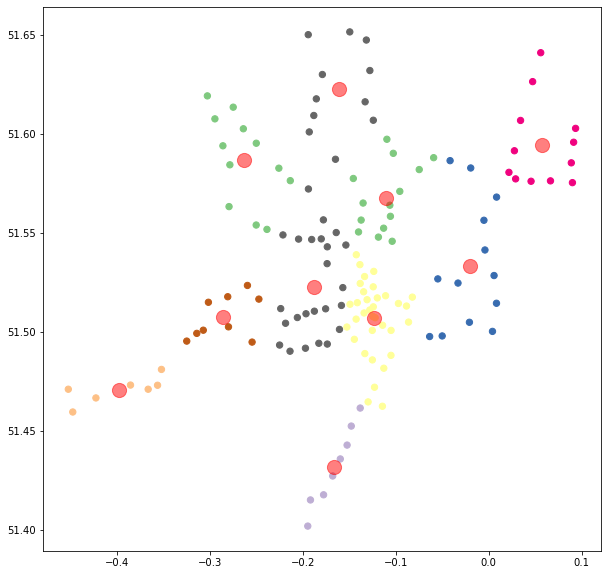Next, we are going to use the KElbowVisualizer from the yellowbrick to pick a number of clusters.

In :
from yellowbrick.cluster import KElbowVisualizer

visualizer = KElbowVisualizer(model, k=(2,12),timings=False)
visualizer.fit(coord)   # Fit the data to the visualizer
visualizer.show()       # Finalize and render the figure

/Users/pan/anaconda3/lib/python3.7/site-packages/sklearn/utils/deprecation.py:143: FutureWarning: The sklearn.metrics.classification module is  deprecated in version 0.22 and will be removed in version 0.24. The corresponding classes / functions should instead be imported from sklearn.metrics. Anything that cannot be imported from sklearn.metrics is now part of the private API.
warnings.warn(message, FutureWarning)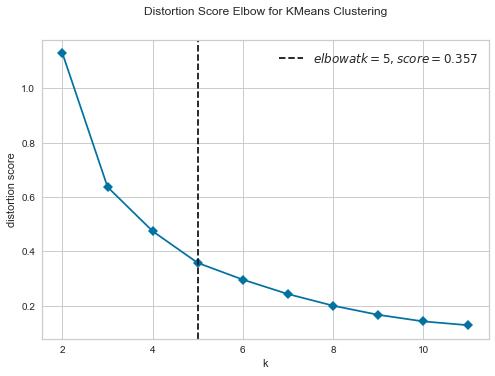Out:
<matplotlib.axes._subplots.AxesSubplot at 0x7fcd1a674cd0>
In :
model = KMeans(n_clusters=5)
model.fit(coord)
clust_pred = model.predict(coord)

plt.scatter(coord[:, 0],
coord[:, 1],
c = clust_pred,
s=plot_size*2,
cmap='Accent')

centers = model.cluster_centers_

plt.scatter(centers[:, 0],
centers[:, 1],
c = 'red',
s=plot_size*10,
alpha=0.5);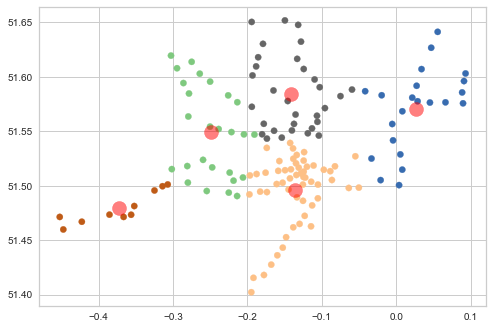These look relalatively sensible upon first sight. Let us now store the cluster memberships into the stations dataframe.

In :
stations['cluster'] = clust_pred
stations

Out:
id latitude longitude name zone cluster
0 1 51.5028 -0.2801 Acton Town 3.0 0
1 8 51.5653 -0.1353 Archway 2.5 4
2 9 51.6164 -0.1331 Arnos Grove 4.0 4
3 10 51.5586 -0.1059 Arsenal 2.0 4
4 11 51.5226 -0.1571 Baker Street 1.0 1
... ... ... ... ... ... ...
139 296 51.5120 -0.2239 White City 2.0 0
140 297 51.5492 -0.2215 Willesden Green 2.5 0
141 303 51.5975 -0.1097 Wood Green 3.0 4
142 301 51.6070 0.0341 Woodford 4.0 2
143 302 51.6179 -0.1856 Woodside Park 4.0 4

144 rows × 6 columns

## Demand Analysis¶

We are lucky enough to have an indication of travel demand patterns in the Night Tube, thanks to the Oyster data that were made available released from Transport for London under their Rolling Origin and Demand Survey (RODS). The entire set of data can be found at:

http://crowding.data.tfl.gov.uk

Before going any further let's load the demand file.

In :
demand = pd.read_csv('datasets/lu_od.csv')
demand

Out:
origin_id dest_id origin_name dest_name demand
0 1 8 Acton Town Archway 0
1 1 9 Acton Town Arnos Grove 1
2 1 10 Acton Town Arsenal 0
3 1 11 Acton Town Baker Street 0
4 1 12 Acton Town Balham 1
... ... ... ... ... ...
20214 43 233 Canning Town Southwark 15
20215 43 23 Canning Town Bermondsey 17
20216 43 41 Canning Town Canada Water 92
20217 43 183 Canning Town North Greenwich 56
20218 43 42 Canning Town Canary Wharf 47

20219 rows × 5 columns

We can see in the above that demands are provided in an "directional" manner - as a flow of customers from one node to another.

To make a better sense of the dataset, we will seek to aggregate these flows into a sum of departures and arrivals for each station.

To do this, we start by creatign two empty dictionaries - to store the total flows. The initial flow value for each station will be zero, and we will be adding flows to the correct place in the dictionary one by one.

We set up these dictionaries by zipping a list of station IDs and an empty array of zeroes - this should be exactly as long as the list of stations. We can do this using the np.zeros(len(stations)) command.

In :
dict_from = dict(zip(stations['id'],np.zeros(len(stations))))
dict_to = dict(zip(stations['id'],np.zeros(len(stations))))


In the next step, we will be going into each node in our set one by one, and we will be summing the flow values on our demand table for the current node, with respect to the origin_id and dest_id columns, respectively.

In :
for node in dict_from:
dict_from[node] = demand.loc[demand['origin_id'] == node, 'demand'].sum()

for node in dict_to:
dict_to[node] = demand.loc[demand['dest_id'] == node, 'demand'].sum()


We can now store these values into our stations dataframe.

In :
stations['tot_departures'] = dict_from.values()
stations['tot_arrivals'] = dict_to.values()
stations

Out:
id latitude longitude name zone cluster tot_departures tot_arrivals
0 1 51.5028 -0.2801 Acton Town 3.0 0 119 603
1 8 51.5653 -0.1353 Archway 2.5 4 252 919
2 9 51.6164 -0.1331 Arnos Grove 4.0 4 76 515
3 10 51.5586 -0.1059 Arsenal 2.0 4 40 350
4 11 51.5226 -0.1571 Baker Street 1.0 1 1586 887
... ... ... ... ... ... ... ... ...
139 296 51.5120 -0.2239 White City 2.0 0 474 407
140 297 51.5492 -0.2215 Willesden Green 2.5 0 187 1091
141 303 51.5975 -0.1097 Wood Green 3.0 4 315 1264
142 301